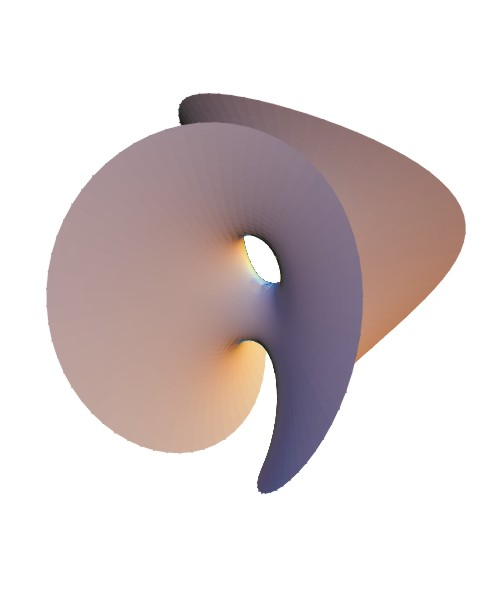Complete minimal surfaces in R3 Francisco J. López & Francisco Martín Publicacions Matematiques, Vol. 43, No. 2, (1999) 341-449.   Full Text: PDF file (1.39 MB). SECTION 1  Preliminaries SECTION 2 Construction of minimal surfaces with polygonal boundary SECTION 3 Gauss map of minimal surfaces SECTION 4 Complete minimal surfaces with bounded coordinate functions SECTION 5 Complete minimal surfaces with finite total curvature SECTION 6 Properly embedded minimal surfacesChen-Gackstatter surface of genus 1. In this paper we review some topics on the theory of minimal surfaces in three dimensional Euclidean space. The study of minimal surfaces in R3 started with Lagrange in 1762. He studied the problem of determining a graph over an open set W in R2, with the least possible area among all surfaces that assume given values on the boundary of W. Mathematics soon realized that here was not only a problem of extraordinary difficulty, but also of unlimited possibilities. In 1776, Meusnier supplied a geometric interpretation of the minimal graph equation: the mean curvature H vanishes. On this premise it has become customary to use the term minimal surface for any surface satisfying H=0, notwithstanding the fact that such surfaces often do not provide a minimum for the area. During the nineteenth century, more discoveries and publications appeared, thanks to the works of Catalan, Bonnet, Serret, Riemann, Weierstrass, Enneper, Schwarz, among others. From the point of view of modern theory, Weierstrass and Enneper's works are specially important. They introduced the so called  Enneper-Weierstrass representation for minimal surfaces, which established a closed relationship between this theory and Complex Analysis. In the middle of the nineteenth century, Plateau observed that minimal surfaces can be physically realized as soap films. So, the problem of determining a minimal surface with fixed topology and bounded by a prescribed Jordan curve is now usually called  Plateau's problem. In this field we place emphasis on the works of Courant, Douglas, Morse, Rado, Schiffman, among others. However, this topic is not covered in this survey. This paper is devoted to some aspects of the theory of complete minimal surfaces. As we will see, completeness has a strong influence on the topology, conformal structure and other geometrical properties of a minimal surface. To be more precise, we include a brief study of: the Gauss map of complete minimal surfaces, complete minimal surfaces with bounded coordinate functions, some of the last achievements about properly embedded minimal surfaces and what is known about complete minimal surfaces with finite total curvature. The aim of this work is not to carry out an exhaustive exposition of these subjects. We only give a summary of the most relevant results or discuss its main applications and related questions. However, we have tried to compile a complete list of references that could help the interested reader to delve more deeply into these subjects. Section 3 is devoted to the Gauss map of minimal surfaces, where we deal with Fujimoto's theorem, which asserts that the plane is the only complete minimal surface in R3 whose Gauss map omits at least five points of the sphere. We include the Osserman-Mo generalization of this theorem.  In Section 4 we study complete minimal surfaces which are bounded as subsets of R3 (Naridashvili's theorem), and some questions related with Calabi's problem for minimal surfaces. In Section 5 (the most extensive) we deal with complete minimal surfaces of finite total curvature. We look at Osserman's classical theorems and the formula of Jorge and Meeks, including a complete list of examples of surfaces of this kind. Our interest lies in: existence and uniqueness theorems for surfaces with critical total curvature (from the point of view of the Jorge-Meeks formula), minimal surfaces with high symmetry group, and nonorientable minimal surfaces. The study of embedded minimal surfaces with finite total curvature is included in Section 5. Finally, in Section 6 we briefly study some of the last achievements in the theory of properly embedded minimal surfaces in R3. Since the discovery of Costa's minimal surface, the study of this area gathered new speed. From Collin's theorem, a properly embedded minimal surface has finite topology if and only if it has finite total curvature, and provided that the number of ends is greater or equal to two. In this section, we review the families of surfaces with three or more ends, placing the emphasis the study of the Costa-Hoffman-Meeks and Hoffman-Meeks families of surfaces. We also include a uniqueness theorem for the Hoffman-Meeks family in terms of its symmetry. The last part of Section 6 is devoted to Meeks' conjecture about properly embedded planar domains. So, we prove the López-Ros theorem and include a summary of the Meeks-Pérez-Ros theorem.

RECENT PAPERS